# 用 Google Colab 免費 GPU 訓練 AI 模型教學

## 用免費的 Google Colab GPU訓練 AI 模型

Google Colab 其實就是一個 Web 版的 Python Runtime 執行環境，屬於 Google Drive 的一種應用程式，使用者界面跟 Jupyter Notebook 長的差不多，用起來也很相似。開始介紹如何利用 Colab GPU 訓練模型，首先進入 Google Colab 後，選擇「NEW PYTHON 3 NOTEBOOK」建立一個新的 ipynb 檔案，如下：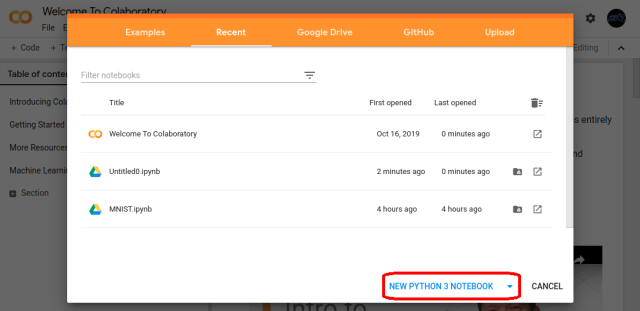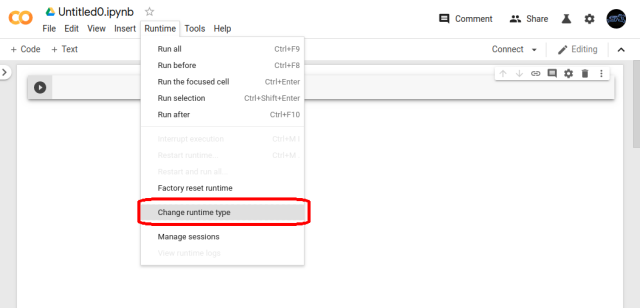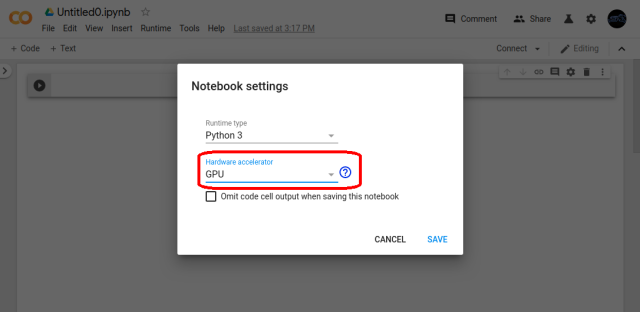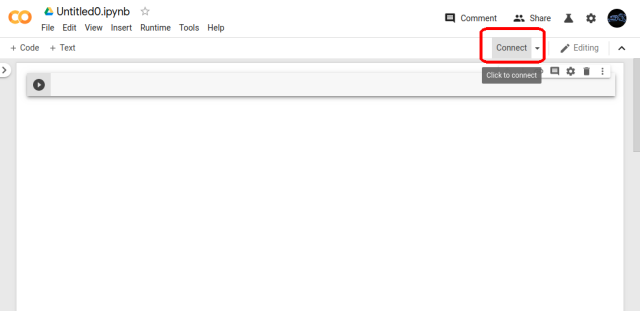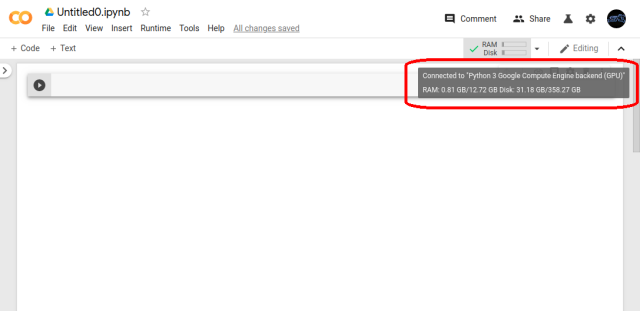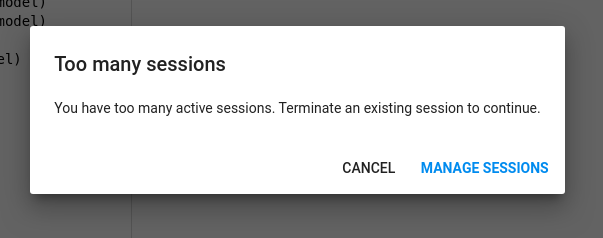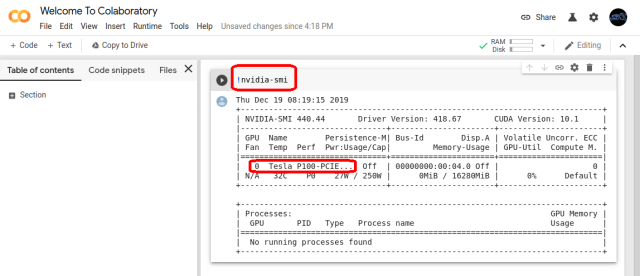## 用 Google Colab GPU 訓練 Model

```import cv2
import numpy as np

from keras.applications.vgg16 import VGG16
from keras.layers import Input
from keras.layers import Flatten
from keras.layers import Dense
from keras.layers import Dropout
from keras.models import Model
from keras.utils import np_utils
from keras.datasets import mnist

epochs = 10
batch_size = 50
row_col = 48

# 原始的 MNIST 是 6000 筆 28*28 灰階
(X_train, y_train), (X_test, y_test) = mnist.load_data()
X_train, y_train = X_train[:5000], y_train[:5000]
X_test, y_test = X_test[5000:6000], y_test[5000:6000]
X_train = [cv2.cvtColor(cv2.resize(i, (row_col, row_col)), cv2.COLOR_GRAY2RGB)
for i in X_train]
X_train = np.concatenate([arr[np.newaxis] for arr in X_train]).astype('float32')
X_test = [cv2.cvtColor(cv2.resize(i, (row_col, row_col)), cv2.COLOR_GRAY2RGB)
for i in X_test]
X_test = np.concatenate([arr[np.newaxis] for arr in X_test]).astype('float32')

X_train = X_train / 255
X_test = X_test / 255

y_train_ohe = np_utils.to_categorical(y_train, 10)
y_test_ohe = np_utils.to_categorical(y_test, 10)

return (X_train, y_train_ohe), (X_test, y_test_ohe)

base_network = VGG16(include_top=False, weights='imagenet', input_shape=(row_col, row_col, 3))

# 凍結預設的參數
for layer in base_network.layers:
layer.trainable = False

# 接上自行定義的全連結層
model = Flatten()(base_network.output)
model = Dense(4096, activation='relu', name='full_connect_1')(model)
model = Dense(4096, activation='relu', name='full_connect_2')(model)
model = Dropout(0.5)(model)
model = Dense(10, activation='softmax', name='prediction')(model)
model = Model(base_network.input, model, name='my_model')

return model

# 載入模型
model.summary()

# 載入訓練資料
(x_train, y_train_ohe), (x_test, y_test_ohe) = load_data()
print('Train Size:', x_train.shape)
print('Test Size:', x_test.shape)

#定義 loss function
from keras.optimizers import SGD
sgd = SGD(lr=0.05, decay=1e-5)
model.compile(loss='categorical_crossentropy', optimizer=sgd, metrics=['accuracy'])

# 開始訓練
model.fit(x_train, y_train_ohe, validation_data=(x_test, y_test_ohe), epochs=epochs, batch_size=batch_size)

# 輸出結果
print('Train Acc:', model.evaluate(x_train, y_train_ohe))
print('Test Acc:', model.evaluate(x_test, y_test_ohe))
```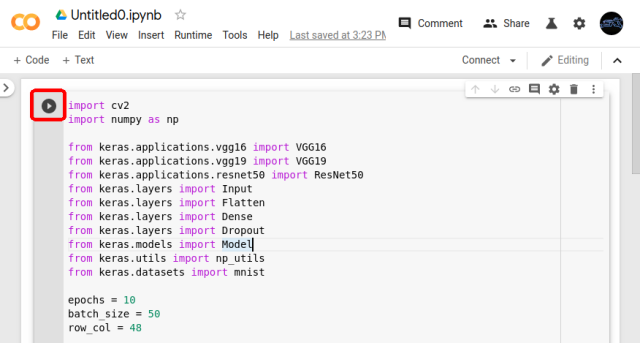Python 執行的過程會顯示在底下，程式執行流程大致如下：

• 從網路下載 Keras 提供的 VGG16 Model
• 凍結 VGG Model 已經訓練好的參數
• 產生兩層 Full Connection 接在原本 VGG Model 的後面
• 輸出接上 Dropout 與 Softmax 層
• 載入 MNIST Dataset 區分 5,000 筆訓練 + 1,000 筆測試
• 跑 10 次 epochs 取得訓練結果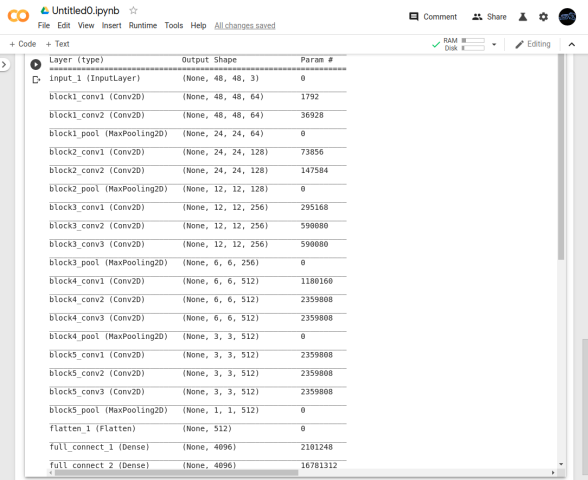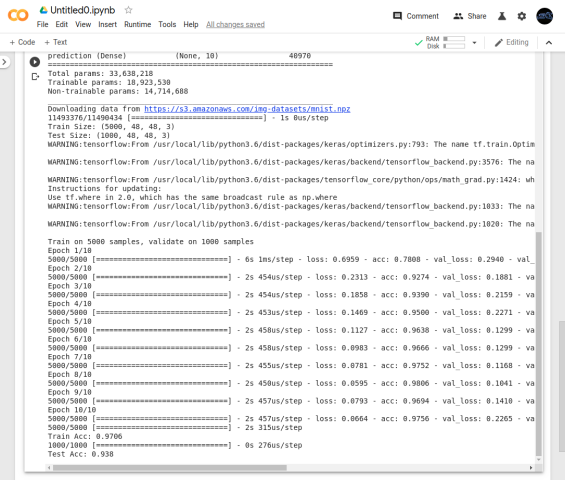Colab 有時候訓練跑很久會被中斷，詳細會被中斷的邏輯我也不清楚，我只有發現 Browser 在背景是很容易斷的。免費的不能要求太多囉 (笑)，基本上 16GB 的 GPU 如果想要訓練太複雜的模型也是會不夠用的，最後也只能 Good Luck 囉，如果訓練成功以後記得按讚分享加訂閱。下次見～